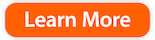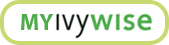Every Math Component Students Need to Review Before the PSAT

With the PSAT just around the corner, now is the time to hone your mathematical skills so you are up-to-date on what’s being asked on the exam. Whether you consider yourself a numbers person or not, it’s a good idea to review what the PSAT will test, so you know how to study.

Although the College Board mentions that preparing in advanced is not required, review sessions can help students feel more confident and reinforce ideas learned in class. Keep reading to learn more about what to expect on the PSAT Math Test so that you can check off every item on your test prep to-do list!

The Basics
The math sections of the PSAT are designed to measure the mathematical skills that you will use in college, your career, and/or your personal life. The exam includes two formats of questions, multiple choice and grid-ins. The PSAT math section is also divided into a Calculator and No Calculator session. There are several topics covered in the math section of the PSAT including Heart of Algebra, Problem & Data Analysis, and the Passport to Advanced Mat.

Heart of Algebra
This section assesses a student’s analytical skills and their ability to fluently solve and create linear equations and inequalities. Some problems in this section may be straightforward while others will pose challenges of strategy and understanding. The questions in this section include both multiple-choice questions and student-produced responses.

Many Heart of Algebra questions will ask students to do one or more of the following: define at least one variable that represents quantities in question, write at least one equation, expression, inequality, or function that represents the relationship in question, or solve an equation and interpret the solution in terms of what the question is asking.

Example:Problem & Data Analysis
This portion of the math section of the PSAT gauges the test taker’s ability to understand and apply quantitative reasoning about ratios, rates, and proportional relationships. Some questions present information about the relationship between two variables in a graph, scatterplot or table. This section will also question you on your understanding of essential concepts in statistics, as well as assessing your conceptual meaning of standard deviation (not to calculate it).

Example:According to the College Board, Passport to Advanced Math questions focus on “the ability to work with and analyze more complex equations. The questions may require you to demonstrate procedural skill in adding, subtracting, and multiplying polynomials and in factoring polynomials” (2019). This section of the examination tests topics that are important for students to master prior to studying more complex levels of mathematics.

Some content that may be covered on this section of the exam includes creating a quadratic or exponential function, determining the most suitable form of an expression, creating equivalent expressions involving rational exponents, and creating an equivalent form of an algebraic expression.

A full list of content covered can be found here.

Example: